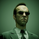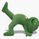TradingView10015 views
This implements the Even Better Sinewave indicator as described in the book Cycle Analysis for Traders by John F. Ehlers.
In the example I used 36 as the cycle to be analyzed and a second cycle with a shorter period, 9, the larger period tells where the dominant cycle is heading, and the faster cycle signals entry/exit points and reversals.
Open-source script

In true TradingView spirit, the author of this script has published it open-source, so traders can understand and verify it. Cheers to the author! You may use it for free, but reuse of this code in a publication is governed by House Rules. You can favorite it to use it on a chart.

Want to use this script on a chart?
```// Madrid : 09/Jun/2015 21:09 : Even Better Sinewave : 1.0
// This implements the Even Better Sinewave indicator
// Ref. Cycle Analysis for Traders by John F. Ehlers.
//

study("Madrid Sinewave", shorttitle="MSineWave")
Duration = input(36)
src = close

OB = 0.85, OS = -0.85
PI = 3.14159265358979

deg2rad( deg ) =>
deg*PI/180.0

lowerBand = input(9)
ssFilter( price, lowerBand ) =>
angle = sqrt(2)*PI/lowerBand
a1= exp(-angle)
b1 = 2*a1*cos(angle)
c2 = b1
c3 = -a1*a1
c1 = 1 - c2 -c3
filt = c1*(price + nz(price))/2 + c2*nz(filt) + c3*nz(filt)

// HighPass filter cyclic components whose periods are shorter than Duration input
x = src
angle = deg2rad(360)/Duration
alpha1 = ( 1-sin(angle) ) / cos(angle)
HP = 0.5*(1+alpha1)*(x-x) + alpha1*nz(HP,0)

// Smooth with a Super Smoother Filter
Filt = ssFilter( HP, lowerBand )

Wave = ( Filt + nz(Filt,0) + nz(Filt,0) ) / 3
Pwr = ( Filt*Filt + nz(Filt,0)* nz(Filt,0) +  nz(Filt,0)* nz(Filt,0) ) /3

// Normalize the Average Wave to Square Root of the Average Power
sineWave = Wave / sqrt(Pwr)

// Output
sineWaveColor = sineWave>OB?green
:  sineWave<OS?red
:  change(sineWave)>0?green
:  red

plot( sineWave, color=sineWaveColor, linewidth=3 )
plot( sineWave, color=sineWaveColor, linewidth=1, style=histogram )

hline(0, color=silver, linestyle=dotted)

```

## CommentsVery interesting
Do you have any example how using it? and di you use 2 different TF or lenght?
thx
ReplyIncredible! I have recently become a big fan of John Ehlers. Been making money on binary options with indicators coded by the excellent programmers we have in TV community. Thanks.
ReplyWow...! This indicator work well for any TF.
Thank you for your contribution.
Madrid, can you add a buy/sell signal button...?
ReplyExcellent work, thank you. Only sinewave signal available for what I can see so far.
Reply